< | Next >

## What is Break Even Point?

Break even point ( BEP) is the point where the revenue is equal to total cost. At BEP, the company makes neither profit nor loss. This point is important to determine the price of a product such that the company still gains net profit. In this tutorial, we will use MS Excel Goal Seek to find the price at certain BEP as well as online interactive BEP calculator . Alternatively, you may also download the spreadsheet here . Before that, let me refresh you on how to compute Break Even Point (BEP) and the terminologies.

Your company may have fixed cost,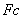such as worker salary, building operation and maintenance, machine and equipments that you must pay every month regardless how much sales that your company can get. Aside from the fixed cost, there is also variable cost,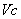such as materials, energy supply (oil or electricity), and worker wages that depend on the production or sales. Higher sales, you may want to produce more and eventually paid higher variable cost.

Let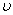be variable cost/ unit and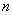is the total sales. We can compute the variable cost as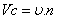and the total cost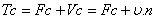. Your company revenue is depend on the total salesand the price of the product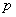, or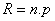.

The break event point happens when the total cost is equal to revenue, or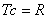which is equivalent to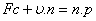or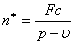(Equation 1)

Thus, the break-even point price is obtained from revenue line at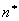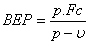(Equation 2)

In the next section , I will explain how to compute BEP using MS Excel. For interactive online break event analysis calculator, click here